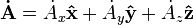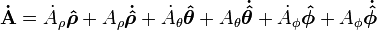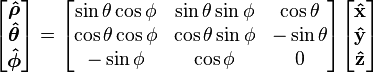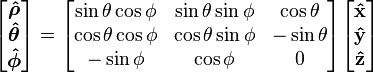# Vector fields in cylindrical and spherical coordinates

I am reading the Wikipedia entry http://en.wikipedia.org/wiki/Vector_fields_in_cylindrical_and_spherical_coordinates" [Broken]. There, in particular I see this:

Time derivative of a vector field

To find out how the vector field A changes in time we calculate the time derivatives. In cartesian coordinates this is simply:However, in spherical coordinates this becomes:So, I wonder, what is the rationale behind assuming that x,y,z are time-independent but $$\rho, \theta,\phi$$ suddenly depend on time even if the realtions between the two are time-independent? Can someone help me here?

Last edited by a moderator:

The basis vectors in x,y,and z-direction stay the same when moving but the basis vectors in $$\rho, \theta, \phi$$ direction change.

For example consider a vector v = (r,0,0) in spherical coordinates, then you have:
$$v = r \hat{\rho}$$
$$\dot{v} = \dot{r} \hat{\rho} + r \dot{\hat{\rho}}$$

The basis vectors in x,y,and z-direction stay the same when moving but the basis vectors in $$\rho, \theta, \phi$$ direction change.

But who is moving and why? Say, my vector vector field is the electric field. What is moving?

Oh I see what you mean, the whole field is not moving. I should have said "changing" instead of "moving".

The wikipedia page just describes how any vector within the field changes over time.

The wikipedia page just describes how any vector within the field changes over time.

There are no vectors "within the field". There is a field, that is a vector-valued function of space and time coordinates. This is the definition of a vector field.

I have no idea what Wikipedia describes. Certainly not what it claims to describe.

Okay, well the page is clearly just describing vectors in cartesian, cilindrical and spherical coordinates, and their time derivatives.

the page should probably be called:
"Vector [STRIKE]fields[/STRIKE] spaces in cylindrical and spherical coordinates"

The name is not important. What is important is the fact that there are no dots over x,y,z but there are dots over $$\rho,\theta,\phi$$, what does not makes sense at all. Either something is moving or not. And when something is moving, then it is moving both in Cartesian and in spherical coordinates. And if someone copied some formulas from some other place that is discussing rotating frames, then it should be said so.

Or, perhaps, I am dumb and Wikipedia is smart. So, maybe someone can explain these things to me, please! Again: what is it that is moving? And why this something is moving in spherical coordinates, but not in Cartesian coordinates?

It is changing in Cartesian coordinates as well, that is why there are dots above the capital A's. There are dots over some of the $$\rho,\theta,\phi$$ because of the use of the product rule when differentiation the position vector.

(in Cartesian coordinates only the coefficients in front of the basisvectors change, in cylindrical and spherical coordinates the coefficients and the basis vectors change)

(in Cartesian coordinates only the coefficients in front of the basisvectors change, in cylindrical and spherical coordinates the coefficients and the basis vectors change)

Why so? The relation between the Cartesian and spherical coordinates:does not involve time. $$\rho,\theta,\phi$$ are supposed to be coordinates of what?

$$\mathbf{\dot{A}} = \dot{A_x} \mathbf{\hat x} + A_x \mathbf{ \dot{\hat{ x}}} + \dot{A_y} \mathbf{\hat y} + A_y \mathbf{\dot{\hat{ y}}} + \do{ A_z} \mathbf{\hat z} + A_y \mathbf{\dot{\hat{ y}}} = \dot{A_x} \mathbf{\hat x} + 0 + \dot{A_y} \mathbf{\hat y} + 0 + \do{ A_z} \mathbf{\hat z} + 0 = \dot{A_x} \mathbf{\hat x} + \dot{A_y} \mathbf{\hat y} + \do{ A_z} \mathbf{\hat z} + = \dot{ A_r} \boldsymbol{\hat{r}} + A_r \boldsymbol{\dot{\hat{ r}}} + \dot{ A_\theta} \boldsymbol{\hat{\theta}} + A_\theta \boldsymbol{\dot{\hat{\theta}}} + \dot {A_z} \boldsymbol{\hat{ z}} + A_z \boldsymbol{\dot{\hat{z}}}$$

$$x,y,z$$ are supposed to be coordinates of what? $$\rho,\theta,\phi$$ are supposed to be coordinates of what?

Why so? The relation between the Cartesian and spherical coordinates:does not involve time. $$\rho,\theta,\phi$$ are supposed to be coordinates of what?

The $$\hat{\rho},\hat{\theta},\hat{\phi}$$ are basis vectors. This expression shows the relation between Cartesian basis vectors and spherical basis vectors.

Every vector in the space is a linear combination of $$\hat{\rho},\hat{\theta},\hat{\phi}$$.

$$A_x, A_y, A_z$$ are the coordinates

$$A_x, A_y, A_z$$ are the coordinates

Coordinates of what? Of a vector? Which vector? Where is this vector located? Is this location changing? If so, with respect to what it is changing?

Yes coordinates of a vector, just any vector in the vector space. This vector can be described in Cartesian coordinates or in Spherical coordinates. The wikipedia page shows what the derivative of such a vector would be when written in Cartesian, cylindrical or spherical coordinates.

Say we have a vector , v, in Cartesian coordinates its coordinates are (a,b,c) and in spherical coordinates they are (d,e,f).
This means that we have:
$$v = a \hat{x}+ b \hat{y} +c \hat{z}$$
and
$$v = d \hat{\rho} + e \hat{\phi} + f \hat{\theta}$$